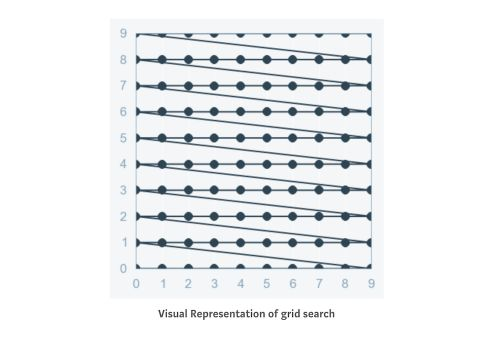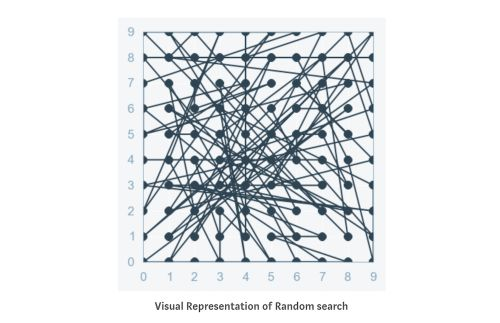Grid Search and Randomized Search are the two most popular methods for hyper-parameter optimization of any model. In both cases, the aim is to test a set of parameters whose range has been specified by the users, and observe the outcome in terms of the metric used (accuracy, precision…).

For a Decision Tree, we would typically set the range of parameters to look for to :

• criterion: Gini or entropy
• max_depth: between 5 and 50
• min_samples_split: between 2 and 5

However, the way the parameters are tested is quite different between Grid Search and Randomized Search.

# Grid Search

In GridSearch, we try every combination of the set of parameters defined above. This means that we will test the following combinations for example :

 Criterion Max Depth Min Samples Split Gini 5 2 Gini 5 3 Gini 5 4 Gini 5 5 Gini 10 2 Gini 10 3 Gini 10 4 Gini 10 5 .. .. .. Entropy 50 5

We can visually represent the grid search on 2 features as a sequential way to test, in order, all the combinations :As you might guess, grid search does not scale well. There is a huge number of combinations we end up testing for just a few parameters. For example, if we have 4 parameters, and we want to test 10 values for each parameter, there are : $$10 \times 10 \times 10 \times 10 = 10'000$$ combinations possible.

Grid search is implemented in scikit-learn under the name of GridSearchCV (for cross validation) :

from sklearn.model_selection import GridSearchCV

param_grid = [
'n_estimators': [3, 10, 30],
'max_features': [2, 4, 6, 8],
'bootstrap' : [True, False]
]

rf = RandomForestRegressor()

grid_search = GridSearchCV(rf, param_grid, cv=5, scoring='mean_squared_error', return_train_score=True)

grid_search.fit(X_val, y_val)


To optimize the hyper-parameters, we tend to use a validation set (if available) to limit the overfitting on the train set.

# Randomized Search

Randomized Search follows the same goal. However, we won’t test sequentially all the combinations. Instead, we try random combinations among the range of values specified for the hyper-parameters. We initially specify the number of random configurations we want to test in the parameter space.

The main advantage is that we can try a broader range of values or hyperparameters within the same computation time as grid search, or test the same ones in much less time. We are however not guaranteed to identify the best combination since not all combinations will be tested.The implementation in scikit-learn is also straight forward :

from sklearn.model_selection import RandomizedSearchCV

param_grid = [
'n_estimators': [3, 10, 30],
'max_features': [2, 4, 6, 8],
'bootstrap' : [True, False]
]

rf = RandomForestRegressor()

rnd_search = RandomizedSearchCV(rf, param_grid, cv=5, scoring='mean_squared_error', return_train_score=True)

rnd_search.fit(X_val, y_val)


Conclusion : There is a tradeoff to make between the guarantee to identify the best combination of parameters and the computation time. A simple trick could be to start with a randomized search to reduce the parameters space and then launch a grid search to select the optimal features within this space.

Categories:

Updated: